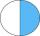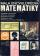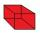# Examples of equations for 6th grade (11y)

#### Number of problems found: 286

• Simple equation 324 = n • 27, solve for n
• Reciprocal equation 3Solve reciprocal equation: 1/2 + 2/3=1/x
• RootThe root of the equation ? is (equal or greater or less than zero)? ?
• Wood dividingWhich equation calculates the number of 1/3-foot pieces that can be cut from a piece of wood that is 7 feet long?
• EquationSolve equation and check the result: 1.4x - 3/2 + x - 9,8 = x + 0,4/3 - 7 + 1,6/6
• Star equationWrite digits instead of stars so that the sum of the written digits is odd and is true equality: 42 · ∗8 = 2 ∗∗∗
• DenominatorCalculate the missing denominator x: ?
• NumberCalculate the integer number which, divided by 34 gives 10 and the rest 25.
• ExpressionIf it is true that ? is:
• 15 numberWhat number is smaller (greater) by 15 than its half?
• Unknown number 11That number increased by three equals three times itself?
• TimeageSeven times of my age is 8 less than the largest two-digit number. How old I am?
• BookTo number pages of the thick book was used 4201 digits. How many pages has this book?
• The quotientThe quotient of g and 55 is the same as 279. What is g?
• NumberDetermine unknown number if you know that difference between five times and triple of number is 42.
• Six te 2If 3t-7=5t, then 6t=
• Have solutionThe sum of four consecutive even numbers is 92. Determine these numbers.
• QuizTested student answered correctly on a 4/ 8 questions. Wrong answers was 16. How many questions answered?
• Missing numberBlank +1/6 =3/2 find the missing number
• Special cubeCalculate the edge of cube, if its surface and its volume is numerically equal number.

Do you have an interesting mathematical word problem that you can't solve it? Submit a math problem, and we can try to solve it.

We will send a solution to your e-mail address. Solved examples are also published here. Please enter the e-mail correctly and check whether you don't have a full mailbox.

Please do not submit problems from current active competitions such as Mathematical Olympiad, correspondence seminars etc...

Do you have a linear equation or system of equations and looking for its solution? Or do you have quadratic equation?